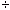SEARCH HOMEMath Central Quandaries & QueriesQuestion from fatima, a student: how can i change a fraction into a decimal and how do i change a decimal into a fraction................ anther question please what does making a profit have to do with percentage change!!!!!!Hi Fatima,

To convert a common fraction like 3/20 into a decimal you divide the denominator into the numerator so, since 320 = 0.15, 3/20 = 0.15.

To see how to convert a decimal into a common fraction look at the response we sent to Andres a while ago.

Suppose that you produce an item you want to sell and it cost you $10.00 to produce it. You want to sell it for more than$10.00 so you will make a profit. Suppose you decide to sell it for $15.00 so that you make a profit of$5.00. Many times you see this markup or profit expressed as a percentage of what it cost you to produce the item. In this case the profit is $5.00 and the cost was$10.00 so the percentage markup is

5/10100 = 50%.

You might see this as a percentage change from your cost ($10.00) to your customer's cost ($15.00).

PennyMath Central is supported by the University of Regina and The Pacific Institute for the Mathematical Sciences.